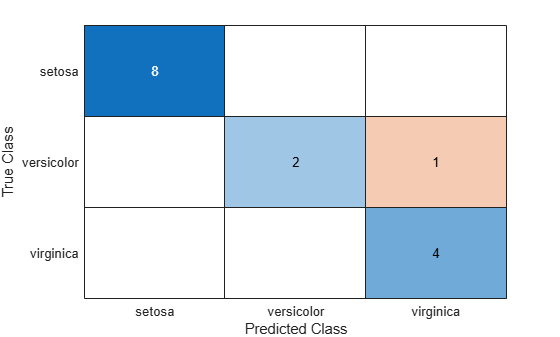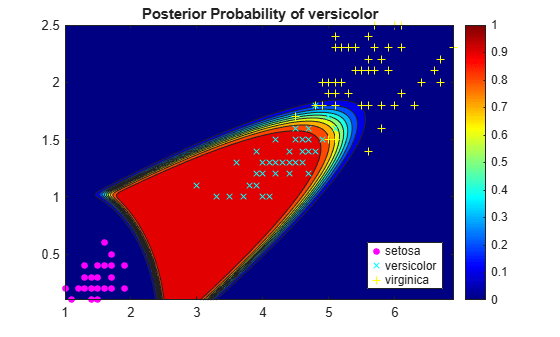# predict

Predict labels using discriminant analysis classification model

## Syntax

``label = predict(Mdl,X)``
``````[label,score,cost] = predict(Mdl,X)``````

## Description

````label = predict(Mdl,X)` returns a vector of predicted class labels for the predictor data in the table or matrix `X`, based on the trained discriminant analysis classification model `Mdl`.```
``````[label,score,cost] = predict(Mdl,X)``` also returns: A matrix of classification scores (`score`) indicating the likelihood that a label comes from a particular class. For discriminant analysis, scores are posterior probabilities.A matrix of expected classification cost (`cost`). For each observation in `X`, the predicted class label corresponds to the minimum expected classification cost among all classes. ```

## Input Arguments

expand all

Discriminant analysis classification model, specified as a `ClassificationDiscriminant` or `CompactClassificationDiscriminant` model object returned by `fitcdiscr`.

Predictor data to be classified, specified as a numeric matrix or table.

Each row of `X` corresponds to one observation, and each column corresponds to one variable. All predictor variables in `X` must be numeric vectors.

• For a numeric matrix, the variables that compose the columns of `X` must have the same order as the predictor variables that trained `Mdl`.

• For a table:

• `predict` does not support multi-column variables and cell arrays other than cell arrays of character vectors.

• If you trained `Mdl` using a table (for example, `Tbl`), then all predictor variables in `X` must have the same variable names and data types as those that trained `Mdl` (stored in `Mdl.PredictorNames`). However, the column order of `X` does not need to correspond to the column order of `Tbl`. `Tbl` and `X` can contain additional variables (response variables, observation weights, etc.), but `predict` ignores them.

• If you trained `Mdl` using a numeric matrix, then the predictor names in `Mdl.PredictorNames` and corresponding predictor variable names in `X` must be the same. To specify predictor names during training, see the `PredictorNames` name-value pair argument of `fitcdiscr`. `X` can contain additional variables (response variables, observation weights, etc.), but `predict` ignores them.

Data Types: `table` | `double` | `single`

## Output Arguments

expand all

Predicted class labels, returned as a categorical or character array, logical or numeric vector, or cell array of character vectors.

`label`:

• Is the same data type as the observed class labels (`Y`) that trained `Mdl`. (The software treats string arrays as cell arrays of character vectors.)

• Has length equal to the number of rows of `X`.

Predicted class posterior probabilities, returned as a numeric matrix of size `N`-by-`K`. `N` is the number of observations (rows) in `X`, and `K` is the number of classes (in `Mdl.ClassNames`). `score(i,j)` is the posterior probability that observation `i` in `X` is of class `j` in `Mdl.ClassNames`.

Expected classification costs, returned as a matrix of size `N`-by-`K`. `N` is the number of observations (rows) in `X`, and `K` is the number of classes (in `Mdl.ClassNames`). `cost(i,j)` is the cost of classifying row `i` of `X` as class `j` in `Mdl.ClassNames`.

## Examples

expand all

Load Fisher's iris data set. Determine the sample size.

```load fisheriris N = size(meas,1);```

Partition the data into training and test sets. Hold out 10% of the data for testing.

```rng(1); % For reproducibility cvp = cvpartition(N,'Holdout',0.1); idxTrn = training(cvp); % Training set indices idxTest = test(cvp); % Test set indices```

Store the training data in a table.

```tblTrn = array2table(meas(idxTrn,:)); tblTrn.Y = species(idxTrn);```

Train a discriminant analysis model using the training set and default options.

`Mdl = fitcdiscr(tblTrn,'Y');`

Predict labels for the test set. You trained `Mdl` using a table of data, but you can predict labels using a matrix.

`labels = predict(Mdl,meas(idxTest,:));`

Construct a confusion matrix for the test set.

`confusionchart(species(idxTest),labels)``Mdl` misclassifies one versicolor iris as virginica in the test set.

Load Fisher's iris data set. Consider training using the petal lengths and widths only.

```load fisheriris X = meas(:,3:4);```

Train a quadratic discriminant analysis model using the entire data set.

`Mdl = fitcdiscr(X,species,'DiscrimType','quadratic');`

Define a grid of values in the observed predictor space. Predict the posterior probabilities for each instance in the grid.

```xMax = max(X); xMin = min(X); d = 0.01; [x1Grid,x2Grid] = meshgrid(xMin(1):d:xMax(1),xMin(2):d:xMax(2)); [~,score] = predict(Mdl,[x1Grid(:),x2Grid(:)]); Mdl.ClassNames```
```ans = 3x1 cell {'setosa' } {'versicolor'} {'virginica' } ```

`score` is a matrix of class posterior probabilities. The columns correspond to the classes in `Mdl.ClassNames`. For example, `score(j,1)` is the posterior probability that observation `j` is a setosa iris.

Plot the posterior probability of versicolor classification for each observation in the grid and plot the training data.

```figure; contourf(x1Grid,x2Grid,reshape(score(:,2),size(x1Grid,1),size(x1Grid,2))); h = colorbar; caxis([0 1]); colormap jet; hold on gscatter(X(:,1),X(:,2),species,'mcy','.x+'); axis tight title('Posterior Probability of versicolor'); hold off```The posterior probability region exposes a portion of the decision boundary.

expand all